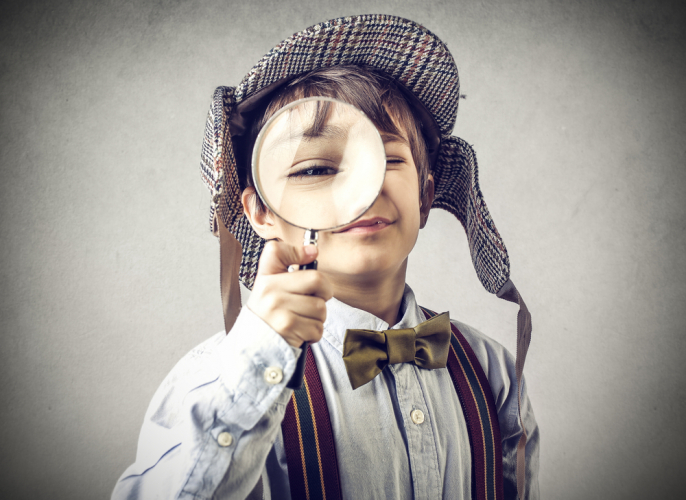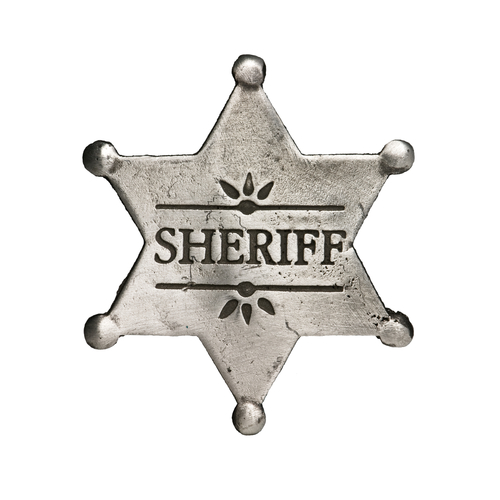# Find the Next Letter and Number Pair in a Sequence

In this worksheet, students will solve sequences involving combined letter and number pairs, with more complex steps, involving addition and forward motion. It will develop students’ problem solving and sequencing skills.Key stage:  KS 2

Curriculum topic:  Verbal Reasoning

Curriculum subtopic:  Letter Connections

Difficulty level:### QUESTION 1 of 10

Hi there code detective, the puzzles are coming in thick and fast!  Are you ready to try something new? These sequences involve both letters and numbers!

The letters and numbers are joined together in a special order.Here is the alphabet for you to use as a reference:

A B C D E F G H I J K L M N O P Q R S T U V W X Y Z

Look at the following letter and number pair sequence:

C6 is to D8 as E10 is to ______?

First, we need to work out how the first letter becomes the second letter.

The letters are moving one place to the right in the alphabet.

Now we need to work out how the first number becomes the second number. This happens by adding two each time.

So we need to add one letter to E and two numbers to 10 to get F12.

C6 is to D8 as E10 is to F12.Let’s try another:

G7 is to K9 as S12 is to ______?

First, we need to work out how the first letter becomes the second letter.

The letters are moving four places to the right in the alphabet.

Now we need to work out how the first number becomes the second number. This is adding two each time.

So we need to add four letters to S and two numbers to 12 to get W14.

G7 is to K9 as S12 is to W14.

In this activity, you will need to be sequence superstar to complete the number and letter pairs.

Remember to use the alphabet line to help you work out the code.

Here are two groups of letter and number sequences. Each group follows the same special sequence, but with different letters and numbers.

U39 is to X43 as Y44 is to _____ ?A  B  C  D  E  F  G  H  I  J  K  L  M  N  O  P  Q  R  S  T  U  V  W  X  Y  Z

Here are two groups of letter and number sequences. Each group follows the same special sequence, but with different letters and numbers.

H76 is to M83 as X61 is to ______ ?

 A  B  C  D  E  F  G  H  I  J  K  L  M  N  O  P  Q  R  S  T  U  V  W  X  Y  Z

Here are two groups of letter and number sequences. Each group follows the same special sequence, but with different letters and numbers.

R75 is to B83 as L52 is to ______ ?A  B  C  D  E  F  G  H  I  J  K  L  M  N  O  P  Q  R  S  T  U  V  W  X  Y  Z

Here are two groups of letter and number sequences. Each group follows the same special sequence, but with different letters and numbers.

Q67 is to Z77 as W35 is to ______ ?

 A  B  C  D  E  F  G  H  I  J  K  L  M  N  O  P  Q  R  S  T  U  V  W  X  Y  Z

Here are two groups of letter and number sequences. Each group follows the same special sequence, but with different letters and numbers.

S63 is to B71 as E91 is to ______ ?A  B  C  D  E  F  G  H  I  J  K  L  M  N  O  P  Q  R  S  T  U  V  W  X  Y  Z

Here are two groups of letters and numbers. Each group follows the same special sequence, but with different letters and numbers.

F44 is to O51  as Z65 is to ______ ?

 A  B  C  D  E  F  G  H  I  J  K  L  M  N  O  P  Q  R  S  T  U  V  W  X  Y  Z

Here are two groups of letter and number sequences. Each group follows the same special sequence, but with different letters and numbers.

W78 is to B84 as R92 is to ______ ?A  B  C  D  E  F  G  H  I  J  K  L  M  N  O  P  Q  R  S  T  U  V  W  X  Y  Z

Here are two groups of letter and number sequences. Each group follows the same special sequence, but with different letters and numbers.

V71 is to F81 as U55 is to ______ ?

 A  B  C  D  E  F  G  H  I  J  K  L  M  N  O  P  Q  R  S  T  U  V  W  X  Y  Z

Here are two groups of numbers. Each group follows the same special sequence, but with different numbers.

C59 is to L67 as Y67 is to ______ ?A  B  C  D  E  F  G  H  I  J  K  L  M  N  O  P  Q  R  S  T  U  V  W  X  Y  Z

Here are two groups of letter and number sequences. Each group follows the same special sequence, but with different letters and numbers.

X89 is to D94 as J88 is to ______ ?

 A  B  C  D  E  F  G  H  I  J  K  L  M  N  O  P  Q  R  S  T  U  V  W  X  Y  Z

• Question 1

Here are two groups of letter and number sequences. Each group follows the same special sequence, but with different letters and numbers.

U39 is to X43 as Y44 is to _____ ?A  B  C  D  E  F  G  H  I  J  K  L  M  N  O  P  Q  R  S  T  U  V  W  X  Y  Z
B48
EDDIE SAYS
Hi there, detective! Remember, we are looking for two sequences here.
The letter sequence follows the alphabet forwards three jumps. Don't forget that if you reach the end of the alphabet whilst jumping in a sequence, you start back at the beginning. The number sequence is add four.
• Question 2

Here are two groups of letter and number sequences. Each group follows the same special sequence, but with different letters and numbers.

H76 is to M83 as X61 is to ______ ?

 A  B  C  D  E  F  G  H  I  J  K  L  M  N  O  P  Q  R  S  T  U  V  W  X  Y  Z
C68
EDDIE SAYS
The letter sequence is to make five jumps forwards in the alphabet. The number sequence is to add seven. Great!
• Question 3

Here are two groups of letter and number sequences. Each group follows the same special sequence, but with different letters and numbers.

R75 is to B83 as L52 is to ______ ?A  B  C  D  E  F  G  H  I  J  K  L  M  N  O  P  Q  R  S  T  U  V  W  X  Y  Z
V60
EDDIE SAYS
Getting the hang of it? The letter sequence here is to take ten jumps forwards in the alphabet. The number sequence is to add eight.
• Question 4

Here are two groups of letter and number sequences. Each group follows the same special sequence, but with different letters and numbers.

Q67 is to Z77 as W35 is to ______ ?

 A  B  C  D  E  F  G  H  I  J  K  L  M  N  O  P  Q  R  S  T  U  V  W  X  Y  Z

F45
EDDIE SAYS
Brilliant effort! The letter sequence is to make nine jumps forwards in the alphabet. The number sequence is to add ten.
• Question 5

Here are two groups of letter and number sequences. Each group follows the same special sequence, but with different letters and numbers.

S63 is to B71 as E91 is to ______ ?A  B  C  D  E  F  G  H  I  J  K  L  M  N  O  P  Q  R  S  T  U  V  W  X  Y  Z

N99
EDDIE SAYS
Did you find the missing letter and number? The letter sequence is to make nine jumps forwards in the alphabet. The number sequence is to add eight.
• Question 6

Here are two groups of letters and numbers. Each group follows the same special sequence, but with different letters and numbers.

F44 is to O51  as Z65 is to ______ ?

 A  B  C  D  E  F  G  H  I  J  K  L  M  N  O  P  Q  R  S  T  U  V  W  X  Y  Z
I72
EDDIE SAYS
Super sequencing skills! The letter sequence is to make nine jumps forwards in the alphabet. The number sequence is to add seven.
• Question 7

Here are two groups of letter and number sequences. Each group follows the same special sequence, but with different letters and numbers.

W78 is to B84 as R92 is to ______ ?A  B  C  D  E  F  G  H  I  J  K  L  M  N  O  P  Q  R  S  T  U  V  W  X  Y  Z
W98
EDDIE SAYS
You're doing great, detective! The letter sequence is to make five jumps forwards in the alphabet. The number sequence is to add six.
• Question 8

Here are two groups of letter and number sequences. Each group follows the same special sequence, but with different letters and numbers.

V71 is to F81 as U55 is to ______ ?

 A  B  C  D  E  F  G  H  I  J  K  L  M  N  O  P  Q  R  S  T  U  V  W  X  Y  Z

E65
EDDIE SAYS
The letter sequence here is to make ten jumps forwards in the alphabet. The number sequence is to add ten. Whoosh, you're whizzing through these questions!
• Question 9

Here are two groups of numbers. Each group follows the same special sequence, but with different numbers.

C59 is to L67 as Y67 is to ______ ?A  B  C  D  E  F  G  H  I  J  K  L  M  N  O  P  Q  R  S  T  U  V  W  X  Y  Z

H75
EDDIE SAYS
Are you getting quicker at these?
The letter sequence is to make nine jumps forwards in the alphabet. The number sequence is to add eight. What a lot of leaping!
• Question 10

Here are two groups of letter and number sequences. Each group follows the same special sequence, but with different letters and numbers.

X89 is to D94 as J88 is to ______ ?

 A  B  C  D  E  F  G  H  I  J  K  L  M  N  O  P  Q  R  S  T  U  V  W  X  Y  Z